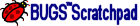Chapter 6. Editing an Aquifer System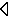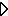## 6.13 Specifying Reaction Rate Data

To edit the rate or rule data for a reaction, the user must bring up the rate data editing page for the reaction. This is the same regardless of the reaction type. First the Form View Reactions Window must be active. Then select the rate data tab. BUGS SCRATCHPAD supports many different types of reactions. Each reaction appears in a different screen. This section illustrates how to enter rate or rule data for each type of a Reaction.

### 6.13.1 Equilibrium Equation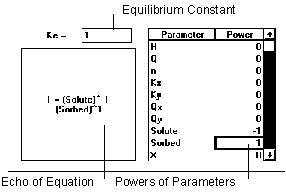Figure 6.11 Equilibrium Reaction

The equilibrium reaction adjusts the values of parameters given in the Stoichiometry relationship (if such adjustment does not cause a parameter to have a negative concentration value) such that the ratios of their values match the equilibrium constant.

### 6.13.2 Equality Equation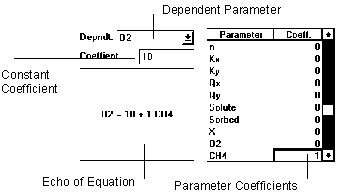Figure 6.12 Equality Reaction

The equality reaction simply sets the dependent parameter to the value specified by the linear combination of parameters and constant.

### 6.13.3 Single Order Reaction Equation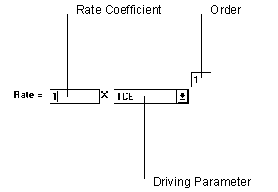Figure 6.13 Single Order Reaction

The single order reaction only uses data from one parameter to determine the rate of reaction.

### 6.13.4 Mixed Ordered Reaction Equation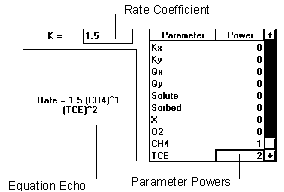Figure 6.14 Mixed Ordered Reaction

The mixed order reaction uses values of multiple parameters to determine the rate of the reaction.

### 6.13.5 Linear Equilibrium Isotherm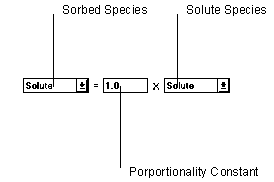Figure 6.15 Linear Equilibrium Isotherm

The linear equilibrium sorption isotherm adjusts parameters using the relationship defined in the Stoichiometry to set the left hand side of the equation equal to the right hand side.

### 6.13.6 Langmuir Equilibrium Isotherm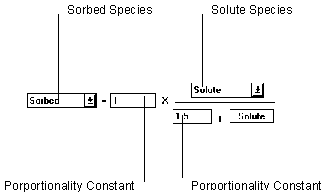Figure 6.16 Langmuir Equilibrium Isotherm

The Langmuir Equilibrium sorption isotherm adjusts parameters using the relationship defined in the Stoichiometry to set the left hand side of the equation equal to the right hand side.

### 6.13.7 Fredulich Equilibrium Isotherm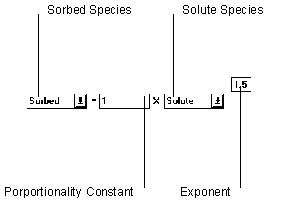Figure 6.17 Fredulich Equilibrium Isotherm

The Fredulich Equilibrium sorption isotherm adjusts parameters using the relationship defined in the Stoichiometry to set the left hand side of the equation equal to the right hand side.

### 6.13.8 Linear Kinetic Isotherm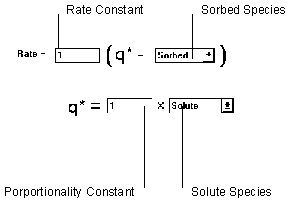Figure 6.18 Linear Kinetic Isotherm

The linear kinetic isotherm computes a target sorbed phase concentration with the linear isotherm relationship. A mass transfer equation is then used to determine the rate of reaction.

### 6.13.9 Langmuir Kinetic Isotherm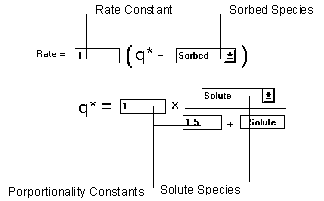Figure 6.19 Langmuir Kinetic Isotherm

The Langmuir Kinetic isotherm uses the Langmuir isotherm relationship to compute a target sorbed phase concentration. A mass transfer equation is then used to determine the rate of reaction.

### 6.13.10 Fredulich Kinetic Isotherm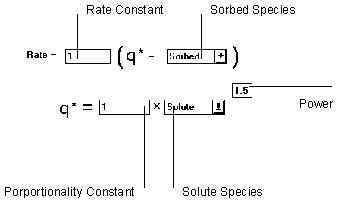Figure 6.20 Fredulich Kinetic Isotherm

The Fredulich Kinetic isotherm uses the Fredulich isotherm relationship to compute a target sorbed phase concentration. A mass transfer equation is then used to determine the rate of reaction.

### 6.13.11 Single Monod Equation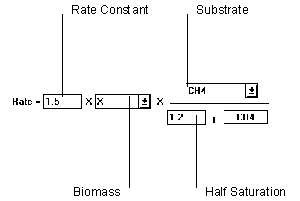Figure 6.21 Single Monod Reaction

The Single Monod equation relies on values from two parameters to determine the rate of reaction. However, the biomass portion can be ignored by setting to "unity".

### 6.13.12 Double Monod Equation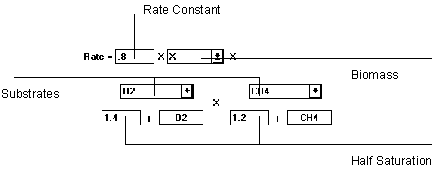Figure 6.22 Double Monod Reaction

The Double Monod equation relies on three parameters to compute the rate of reaction, two substrate and one biomass. If the biomass component is not needed, it can be set to "unity".

### 6.13.13 Competitive Inhibition Equation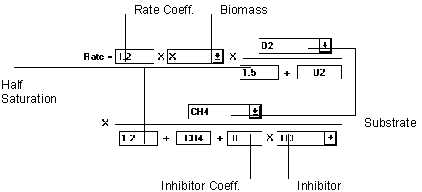Figure 6.23 Competitive Inhibition Reaction

The Competitive Inhibition equation includes a term for a competitive parameter in one of the substrate terms of a double monod relationship. If the biomass term is not needed, it can be set to unity.

### 6.13.14 Mass Transfer Equation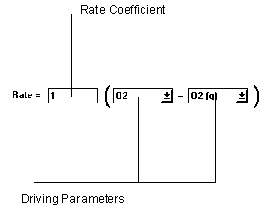Figure 6.24 Mass Transfer Reaction

The mass transfer equation simply uses the difference between two parameter values to compute the rate of reaction.

### 6.13.15 Rule Curve Reaction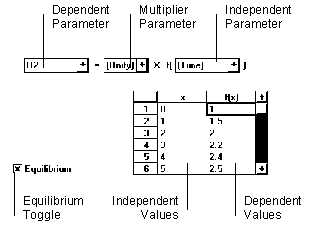Figure 6.25 Rule Curve Reaction

The Rule Curve reaction can be used if all of the above reactions were not appropriate. In addition to accessing the values of parameters, this reaction can also use time as input. For example, an injection schedule could be entered. Independent variables (x) must be entered in acceding order. BUGS SCRATCHPAD uses a simple lookup routine to find values in the list.

Last Updated October 1, 2001